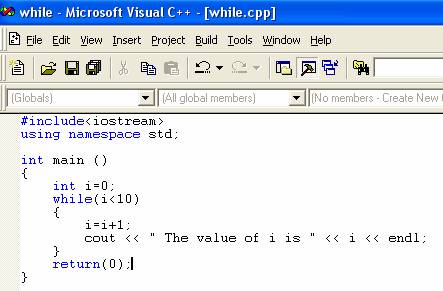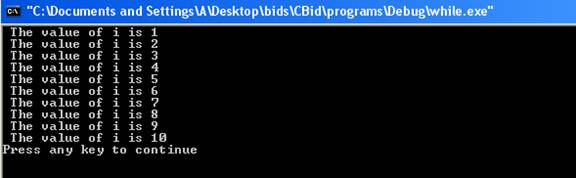The While Loop

The while loop uses a condition to control the execution of the body of the loop. The general form the while loop is: -

while(condition)
{
statement;
}

The condition is an expression which is executed at the beginning of each iteration. If the condition is true then the body of the loop is executed and if it is false loop is terminated and control is transferred to the statement following the while loop. The condition evaluates to a type bool, or to a value of an integer type. If the condition produces the integer, the loop will execute as long as integer is non zero. Non zero integer is converted to type bool as true and if the value of integer is zero it is taken as false. Here is the program which illustrates the working of while loop.The result of the program is: -In the program value of the variable i is incremented by 1 in each iteration. The condition

i<10

checks whether the value of i is less than 10 in every iteration. In every iteration of the loop value of i is incremented by 1 by the statement

i=i+1;

In the last iteration the value of i becomes 10. Then the condition is checked and it returns false, loop is terminated and control is transferred to the statement

return(0);

Go to the previous lesson or proceed to the next lesson